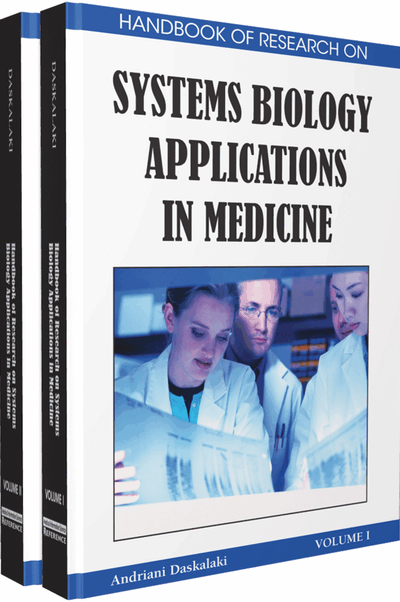# Model Development and Decomposition in Physiology

Isabel Reinecke (Zuse Institute Berlin, Germany) and Peter Deuflhard (Zuse Institute Berlin, Germany)
DOI: 10.4018/978-1-60566-076-9.ch042
Available
\$37.50
No Current Special Offers

## Abstract

In this chapter some model development concepts can be used for the mathematical modeling in physiology as well as a graph theoretical approach for a decomposition technique in order to simplify parameter estimation are presented. This is based on the human menstrual cycle. First some modeling fundamentals are introduced that are applied to the model development of the human menstrual cycle. Then it is shown how a complex mathematical model can be handled if a large number of parameters are used where the parameter values are not known for the most part. A method is presented to divide the model into smaller, disjoint model parts. At the same time, it is shown how this technique works in the case of the human menstrual cycle. The principles for model development and decomposition can be used for other physiological models as well.
Chapter Preview
Top

## Background

First, some principle modeling concepts are introduced that could be useful in the modeling of physiological processes and that are used to construct the complex mathematical model of the human menstrual cycle. The concept of compartmentalization of the considered body parts and how the connections between the compartments can be modeled, for example, via receptor binding and feedback mechanisms, is described. If the biochemical mechanisms are known, simple reaction kinetics can be used and if enzymes catalyze the reaction, simple enzyme kinetics are applied. Taking into account the fact that the different elements of the system influence each other with a certain delay, delay differential equations instead of ordinary differential equations are used.

### Compartmentalization of the Human Body

The human body is not a closed homogeneous system; it consists of organs and tissues etc. in which different processes take place. In order to reduce biological complexity, the body parts that are essential in the processes are extracted and divided into discrete body elements, referred to as compartments (Andersen, 1991) that are interconnected via the shared blood system (Luecke & Wosilait, 1979), here called transport compartment. The characteristics of compartments are that isolated processes take place, but at the same time they can interact with each other. The model formulating the relations between these compartments is called compartmental model (Takeuchi et al., 2007) and this process of organizing the human body in compartments is referred to as compartmentalization which is the concept of pharmacokinetic modeling (Andersen, 1991). More precisely, physiological based compartments are used in this context since the compartments are based on the actual anatomy and physiology (Andersen, 1991).

## Key Terms in this Chapter

Positive and Negative Feedback: If substrates are regulated by other substrates that inhibit or stimulate, we speak of positive and negative feedback, respectively.

Pulse Generator: There are substances that are not released at a constant rate but in pulses. In the hypothalamus there is the GnRH pulse generator responsible for the pulsatile release of GnRH. It is appropriate to choose a stochastic approach for the mathematical model.

Delay Differential Equation: The dynamics are not only dependent on the time point t but on time points that lie in the past.

Compartmentalization: The human body is divided into compartments, open systems that are interconnected.

Michaelis-Menten Mechanism: If reactions are catalyzed by enzymes, then the simplest approach for the mathematical modeling is the Michaelis-Menten mechanism.

Receptor Recycling: The unbound receptors are not active. They become activated when reacting with its ligand. After having accomplished its objective it is not degraded but returns first in an inactivatable state and then in the unbound, activatable state.

Model Decomposition: That is the partition of the mathematical model into disjoint model parts in order to simplify the parameter estimation.

Biphasic Hill Function: At low values the biphasic Hill function is decreasing and at high values increasing.

## Complete Chapter List

Search this Book:
Reset